# 06-240/Final Exam Preparation Forum

Since many of us (including I) don't really know how to use Wiki's, I suggest that we keep the formatting simple: I will post a template at the top of this page, and if you want to add something just click on the "edit", copy the template, and insert your question. Order the questions according to section (i.e. solved/unsolved; whoever created the question must decide if it is solved, and sort it accordingly), with the newest at the top, except for the template question. In general, I wouldn't retype the question if it's from the book because that's tedious and we all have the book.

(By the way, I think you leave a space between lines in the code to make a new line; that is, simply pressing enter once will not make a new line. Also, you can press a button at the top of the editing textbox that lets you put in simple equations.)

## Unsolved Questions

### Question Template

Q: Can someone help me prove: "If an integer$n$ is greater than 2, then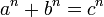$a^n + b^n = c^n$ has no solutions in non-zero integers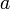$a$,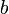$b$, and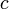$c$."? I had the answer in my head at one point, but the margins of the piece of paper I was working with was too small to fit it.

### Rank of Matrices

Q: Prove that if rankA = 0 (for A with dimension m x n), then A is the zero matrix. (Question is found on p 166 (#3)) Did anyone use transformations in this? My proof relies on rank(L_A) = 0 (left-multiplication transformation)implying L_A is the zero transformation. Is there an easier way?

A: Depends on what you're allowed to assume. If you can use that the rank is equal to the number of linearly independent columns, then clearly all of the columns must be zero (otherwise you have at least one linearly independent vector).

### Determinants

Q : If we can make same matrix with 2n-1 times of row swaps, what does it mean ? Does it mean that determinant is 0 ?

R: Is this related to a question somewhere?

### Exam April/May 2006 #4

Q: Suppose that A, B Є Mmxn(F), and rank(A) = rank (B). Prove that there exist invertible matrices P Є Mmxm(F) and Q Є Mnxn(F) such that B = PAQ.

A(partial): Here is a sketch. If you rref A and B by applying a series of elementary row operation matricies, they will both look similar. That is, they will have a section of 1's and 0's (each 1 is the only number in its column) and then a section of "remaining stuff", and these sections will be the same "size" because their ranks are the same. Then, using the elementary column matrix operations, you can essentially modify the "remaining stuff" as much as you like, by adding multiples of the "nice" columns (with single-1's). These row and column operations can then be grouped nicely and set to be equal to P and Q, which are invertible because products of elementary matricies are invertible.

I know this is very rough, but even if I did have a full answer I wouldn't now how to typeset it.

### Complex Numbers

Q: If 'C' is used in the context of a vector space (as in "define T:C->C"), then should we consider C to be the vector space of C over the field C, or instead C over the field R?

Q: Are we expected to section 5.2 of the textbook? Although the Assignments tell us to read it, we didn't do any questions, or cover it in class.

A: I highly doubt it; hopefully someone will ask Prof. Bar-Natan tomorrow and post the answer here. There were a few other chapters that had sections we never really talked about either (some applications). Addendum: I second this request for a slight narrowing of what the relevant readings are--for instance, can we be more efficient in our reading of chapter 4 somehow?

R: I think that if you want to cut down on Chapter 4, then skipping applications of area (discussed very briefly in class) and determinants of order 2 is the most you can do.

R: What about 4.5, the axiomatic details. It discusses how the determinant is uniquely defined by the three axiomatic properties, but I don't think we did that in class.

## Solved Questions

### Question Template

Q: How many ways are there to get to the nth stair, if at each step you can move either one or two squares up?

A: This question can be easily modeled by the Fibonacci numbers, with the nth number being the ways to get to the nth stair. This is because, to get to the nth stair, you can come only from the n-1th or the n-2th. This is exactly how the Fibonacci numbers are defined; the proof is simple by induction.

### Sec 3.2 Ex. 19

Q: "Let A be an m x n matrix with rank m and B be an n x p matrix with rank n. Determine the rank of AB. Justify your answer." I know how to find that the rank can't be more than m (not much of an accomplishment), but I can't finish it.

A: According to Theorem 3.7(a),(c)&(d)(p.159), I would say rank(AB)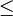$\le$min(m, n).

R: Can we not get any more specific than that?

A: "Let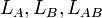$L_A, L_B, L_{AB}$ have their usual meanings. Then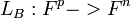$L_B : F^p -> F^n$ is onto. Then we get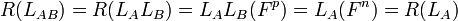$R(L_{AB}) = R(L_A L_B) = L_A L_B (F^p) = L_A (F^n) = R(L_A)$, i.e.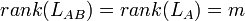$rank(L_{AB}) = rank(L_A) = m$."

### Sec. 3.2 Ex. 21

Q: "Let A be an m x n matrix with rank m. Prove that there exists an n x m matrix B such that AB=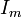$I_m$".

A: "Take any n x m matrix B with rank n. By exercise 19 in the same section rank AB = rank A = m, hence AB is invertible. Let M be the inverse of AB, then (AB)M = A(BM) = I, i.e. BM is the desired matrix."

### Sec. 1.3 Thm 1.3 Proof

Q: In the first paragraph of the proof, it says "But also x + 0 = x , and thus 0'=0." How do we know 0 (that is 0 of V) even exists in W? I understand that we know some zero exists (0'), but not why the zero (0) exists.

A: x is in W as well in V. Thus, x + 0 = x (VS 3).

Reply: Oh I see... now it looks so obvious =/. Thanks.

### Exam April/May 2006 #3(b)

Q: Let T : M3x2(C) -> M2x3(C) be defined as follows. Given A Є M3x2(C), let B be the matrix obtained from A by adding i times the second row of A to the third row of A. Let T(A) = Bt, where Bt is the transpose of B. (Note: Here, i is a complex number such that i2 = -1.) Determine whether the linear transformation T is invertible.

Totally lost on this question :/ Please show some example matrix and how it is transformed as the question asks if possible. I want to see what actually happens to the elements in the matrix rather than the answer (think that would be more important)

A(Matrix Elements): This is my interpretation:

A =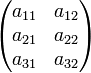$\begin{pmatrix}a_{11}&a_{12}\\a_{21}&a_{22}\\a_{31}&a_{32}\end{pmatrix}$ where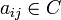$a_{ij} \in C$, then B =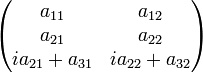$\begin{pmatrix}a_{11}&a_{12}\\a_{21}&a_{22}\\ia_{21}+a_{31}&ia_{22}+a_{32}\end{pmatrix}$.

Therefore, T(A) = Bt is T$\begin{pmatrix}a_{11}&a_{12}\\a_{21}&a_{22}\\a_{31}&a_{32}\end{pmatrix}$=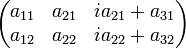$\begin{pmatrix}a_{11}&a_{21}&ia_{21}+a_{31}\\a_{12}&a_{22}&ia_{22}+a_{32}\end{pmatrix}$

R: Thx alot, the matricies are really helpful :)

### Sec. 2.4 Lemma p. 101

Q: In the proof of the lemma, the second line, we have "T(beta) spans R(T) = W". How do we know that R(T) = W? This would be true if dim V = dim W, because then T would be onto, but we can't assume what we're trying to prove.

A: The first line of the Lemma states, "Let T be an invertible linear trans..." So, T is onto(and 1-1), thus "T(beta) spans R(T) = W".

R: Yes. My trouble was with the fact that invertibility implies onto-ness. I thought that if we had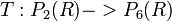$T:P_2 (R)->P_6(R)$, and T(f) = xf, then T would still be invertible since you can 'recover' the f if you were given xf. I guess it makes more sense to not call T invertible in this case, because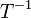$T^{-1}$ is technically only one-to-one over the range of T.

R: T has to be both Onto and 1-1 so that it's invertible. In your example, some of the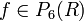$f \in P_6(R)$ will not be 'recovered' because they weren't mapped from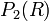$P_2(R)$. Furthermore, T-1 has to map the whole vector space W back to V(as defined on p.99) but not the range only. In other words, if T is 1-1 only,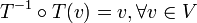$T^{-1} \circ T(v) = v, \forall v\in V$ but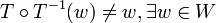$T \circ T^{-1}(w) \neq w, \exists w\in W$, because some T-1(w) are not defined.

R: That nicely rigorizes what I was thinking, and I'm convinced. Thanks.

### Exam April/May 2006 #7

Q: Let T : V -> V and U : V -> V be linear operators on a finite-dimensional vector space V. Assume that U is invertible and T is diagonalizable. Prove that the linear operator UTU-1 = U o T o U-1.

I dont know where or how to start this question ><.

A: I think we need to prove that UTU-1 is diagonalizable instead of proving UTU-1 = U o T o U-1.

I started by letting A = UTU-1, then multiplying U-1 and U to the both sides, we get U-1AU = U-1UTU-1U iff U-1AU = T. Since T is diagonalizable, therefore there exists an invertible matrix Q s.t Q-1TQ = D, where D is a diagonal matrix. Therefore, Q-1(U-1AU)Q = D iff (UQ)-1A(UQ) = D (because U and Q invertible, Q-1U-1 = (UQ)-1), it follows that A is diagonalizable.

### Exam April 2004 #6(a)

Q: Suppose A is an invertible matrix for which the sum of entries of each row is a scalar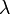$\lambda$. Show that the sum of entries of each row of A-1 is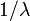$1/\lambda$. (Hint: find an eigenvector for A with eigenvalue$\lambda$.) If A is a diagonal matrix, then it's obvious that the sum of entries of each row is$\lambda$ and the sum of entries of each row of A-1 is$1/\lambda$. I was stuck with a more general invertible matrix.

A: Following the hint, you can see that an eigenvector corresponding to$\lambda$ is (1, 1, 1, ...)*. Therefore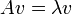$Av=\lambda v$, and rearranging you get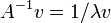$A^{-1}v=1/\lambda v$. Using the same logic as before, you can show that since this$\lambda$ corresponds to a homogeneous system of equations with the same eigenvector v = (1, 1, 1, ...), the sum of each row is equal to$1/\lambda$.

• Just to elaborate on the first part, you are looking for a vector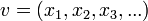$v = (x_1, x_2, x_3, ...)$ so that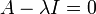$A-\lambda I = 0$. This corresponds to the system: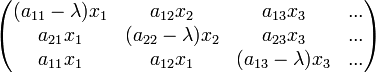$\begin{pmatrix}(a_{11}-\lambda)x_1&a_{12}x_2&a_{13}x_3&...\\a_{21}x_1&(a_{22}-\lambda)x_2&a_{23}x_3&...\\ a_{11}x_1&a_{12}x_1&(a_{13}-\lambda)x_3&...\end{pmatrix}$, and so in each row you can see that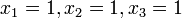$x_1=1, x_2=1, x_3=1$ works because then all the a's in each row add up to$\lambda$.

• Also, does anyone know how to do part (b) of that question? My guess is to make one subspace {0}, the second (t,0,0) and the third (0,r,s) for all t,r,s,. Does that look okay?

R: Thanks. I think the subspaces are {0}, {(t,0,0)} and {(0,s,0)} so that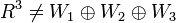$R^3 \neq W_1 \oplus W_2 \oplus W_3$.

R: We need them to add up to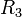$R_3$ though. Anyway, hopefully we won't need to know about direct sums.